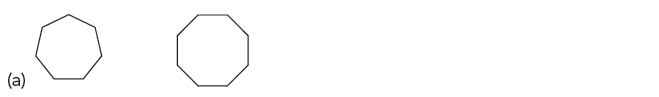# Problem: For each of the following pairs of compounds, identify the compound that would have the higher heat of combustion:

###### Problem Details

For each of the following pairs of compounds, identify the compound that would have the higher heat of combustion:What scientific concept do you need to know in order to solve this problem?

Our tutors have indicated that to solve this problem you will need to apply the Ring Strain concept. You can view video lessons to learn Ring Strain. Or if you need more Ring Strain practice, you can also practice Ring Strain practice problems.

What is the difficulty of this problem?

Our tutors rated the difficulty ofFor each of the following pairs of compounds, identify the c...as medium difficulty.

How long does this problem take to solve?

Our expert Organic tutor, Chris took 1 minute and 49 seconds to solve this problem. You can follow their steps in the video explanation above.

What textbook is this problem found in?

Our data indicates that this problem or a close variation was asked in Organic Chemistry - Klein 1st Edition. You can also practice Organic Chemistry - Klein 1st Edition practice problems.